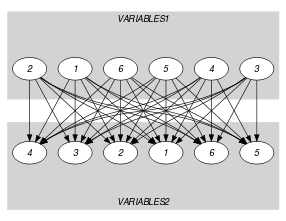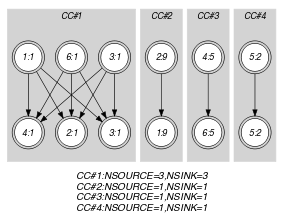## 5.336. same_and_global_cardinality_low_up

Origin
Constraint

$\mathrm{𝚜𝚊𝚖𝚎}_\mathrm{𝚊𝚗𝚍}_\mathrm{𝚐𝚕𝚘𝚋𝚊𝚕}_\mathrm{𝚌𝚊𝚛𝚍𝚒𝚗𝚊𝚕𝚒𝚝𝚢}_\mathrm{𝚕𝚘𝚠}_\mathrm{𝚞𝚙}\left(\mathrm{𝚅𝙰𝚁𝙸𝙰𝙱𝙻𝙴𝚂}\mathtt{1},\mathrm{𝚅𝙰𝚁𝙸𝙰𝙱𝙻𝙴𝚂}\mathtt{2},\mathrm{𝚅𝙰𝙻𝚄𝙴𝚂}\right)$

Arguments
 $\mathrm{𝚅𝙰𝚁𝙸𝙰𝙱𝙻𝙴𝚂}\mathtt{1}$ $\mathrm{𝚌𝚘𝚕𝚕𝚎𝚌𝚝𝚒𝚘𝚗}\left(\mathrm{𝚟𝚊𝚛}-\mathrm{𝚍𝚟𝚊𝚛}\right)$ $\mathrm{𝚅𝙰𝚁𝙸𝙰𝙱𝙻𝙴𝚂}\mathtt{2}$ $\mathrm{𝚌𝚘𝚕𝚕𝚎𝚌𝚝𝚒𝚘𝚗}\left(\mathrm{𝚟𝚊𝚛}-\mathrm{𝚍𝚟𝚊𝚛}\right)$ $\mathrm{𝚅𝙰𝙻𝚄𝙴𝚂}$ $\mathrm{𝚌𝚘𝚕𝚕𝚎𝚌𝚝𝚒𝚘𝚗}\left(\mathrm{𝚟𝚊𝚕}-\mathrm{𝚒𝚗𝚝},\mathrm{𝚘𝚖𝚒𝚗}-\mathrm{𝚒𝚗𝚝},\mathrm{𝚘𝚖𝚊𝚡}-\mathrm{𝚒𝚗𝚝}\right)$
Restrictions
 $|\mathrm{𝚅𝙰𝚁𝙸𝙰𝙱𝙻𝙴𝚂}\mathtt{1}|=|\mathrm{𝚅𝙰𝚁𝙸𝙰𝙱𝙻𝙴𝚂}\mathtt{2}|$ $\mathrm{𝚛𝚎𝚚𝚞𝚒𝚛𝚎𝚍}$$\left(\mathrm{𝚅𝙰𝚁𝙸𝙰𝙱𝙻𝙴𝚂}\mathtt{1},\mathrm{𝚟𝚊𝚛}\right)$ $\mathrm{𝚛𝚎𝚚𝚞𝚒𝚛𝚎𝚍}$$\left(\mathrm{𝚅𝙰𝚁𝙸𝙰𝙱𝙻𝙴𝚂}\mathtt{2},\mathrm{𝚟𝚊𝚛}\right)$ $\mathrm{𝚛𝚎𝚚𝚞𝚒𝚛𝚎𝚍}$$\left(\mathrm{𝚅𝙰𝙻𝚄𝙴𝚂},\left[\mathrm{𝚟𝚊𝚕},\mathrm{𝚘𝚖𝚒𝚗},\mathrm{𝚘𝚖𝚊𝚡}\right]\right)$ $\mathrm{𝚍𝚒𝚜𝚝𝚒𝚗𝚌𝚝}$$\left(\mathrm{𝚅𝙰𝙻𝚄𝙴𝚂},\mathrm{𝚟𝚊𝚕}\right)$ $\mathrm{𝚅𝙰𝙻𝚄𝙴𝚂}.\mathrm{𝚘𝚖𝚒𝚗}\ge 0$ $\mathrm{𝚅𝙰𝙻𝚄𝙴𝚂}.\mathrm{𝚘𝚖𝚊𝚡}\le |\mathrm{𝚅𝙰𝚁𝙸𝙰𝙱𝙻𝙴𝚂}\mathtt{1}|$ $\mathrm{𝚅𝙰𝙻𝚄𝙴𝚂}.\mathrm{𝚘𝚖𝚒𝚗}\le \mathrm{𝚅𝙰𝙻𝚄𝙴𝚂}.\mathrm{𝚘𝚖𝚊𝚡}$
Purpose

The variables of the $\mathrm{𝚅𝙰𝚁𝙸𝙰𝙱𝙻𝙴𝚂}\mathtt{2}$ collection correspond to the variables of the $\mathrm{𝚅𝙰𝚁𝙸𝙰𝙱𝙻𝙴𝚂}\mathtt{1}$ collection according to a permutation. In addition, each value $\mathrm{𝚅𝙰𝙻𝚄𝙴𝚂}\left[i\right].\mathrm{𝚟𝚊𝚕}$ (with $i\in \left[1,|\mathrm{𝚅𝙰𝙻𝚄𝙴𝚂}|\right]$) should be taken by at least $\mathrm{𝚅𝙰𝙻𝚄𝙴𝚂}\left[i\right].\mathrm{𝚘𝚖𝚒𝚗}$ and at most $\mathrm{𝚅𝙰𝙻𝚄𝙴𝚂}\left[i\right].\mathrm{𝚘𝚖𝚊𝚡}$ variables of the $\mathrm{𝚅𝙰𝚁𝙸𝙰𝙱𝙻𝙴𝚂}\mathtt{1}$ collection. Finally, each variable of $\mathrm{𝚅𝙰𝚁𝙸𝙰𝙱𝙻𝙴𝚂}\mathtt{1}$ should be assigned a value of $\mathrm{𝚅𝙰𝙻𝚄𝙴𝚂}\left[i\right].\mathrm{𝚟𝚊𝚕}$ (with $i\in \left[1,|\mathrm{𝚅𝙰𝙻𝚄𝙴𝚂}|\right]$).

Example
$\left(\begin{array}{c}〈1,9,1,5,2,1〉,\hfill \\ 〈9,1,1,1,2,5〉,\hfill \\ 〈\begin{array}{ccc}\mathrm{𝚟𝚊𝚕}-1\hfill & \mathrm{𝚘𝚖𝚒𝚗}-2\hfill & \mathrm{𝚘𝚖𝚊𝚡}-3,\hfill \\ \mathrm{𝚟𝚊𝚕}-2\hfill & \mathrm{𝚘𝚖𝚒𝚗}-1\hfill & \mathrm{𝚘𝚖𝚊𝚡}-1,\hfill \\ \mathrm{𝚟𝚊𝚕}-5\hfill & \mathrm{𝚘𝚖𝚒𝚗}-1\hfill & \mathrm{𝚘𝚖𝚊𝚡}-1,\hfill \\ \mathrm{𝚟𝚊𝚕}-7\hfill & \mathrm{𝚘𝚖𝚒𝚗}-0\hfill & \mathrm{𝚘𝚖𝚊𝚡}-2,\hfill \\ \mathrm{𝚟𝚊𝚕}-9\hfill & \mathrm{𝚘𝚖𝚒𝚗}-1\hfill & \mathrm{𝚘𝚖𝚊𝚡}-1\hfill \end{array}〉\hfill \end{array}\right)$

The $\mathrm{𝚜𝚊𝚖𝚎}_\mathrm{𝚊𝚗𝚍}_\mathrm{𝚐𝚕𝚘𝚋𝚊𝚕}_\mathrm{𝚌𝚊𝚛𝚍𝚒𝚗𝚊𝚕𝚒𝚝𝚢}_\mathrm{𝚕𝚘𝚠}_\mathrm{𝚞𝚙}$ constraint holds since:

• The values 1, 9, 1, 5, 2, 1 assigned to $|\mathrm{𝚅𝙰𝚁𝙸𝙰𝙱𝙻𝙴𝚂}\mathtt{1}|$ correspond to a permutation of the values 9, 1, 1, 1, 2, 5 assigned to $|\mathrm{𝚅𝙰𝚁𝙸𝙰𝙱𝙻𝙴𝚂}\mathtt{2}|$.

• The values 1, 2, 5, 7 and 6 are respectively used 3 ($2\le 3\le 3$), 1 ($1\le 1\le 1$), 1 ($1\le 1\le 1$), 0 ($0\le 0\le 2$) and 1 ($1\le 1\le 1$) times.

Typical
 $|\mathrm{𝚅𝙰𝚁𝙸𝙰𝙱𝙻𝙴𝚂}\mathtt{1}|>1$ $\mathrm{𝚛𝚊𝚗𝚐𝚎}$$\left(\mathrm{𝚅𝙰𝚁𝙸𝙰𝙱𝙻𝙴𝚂}\mathtt{1}.\mathrm{𝚟𝚊𝚛}\right)>1$ $\mathrm{𝚛𝚊𝚗𝚐𝚎}$$\left(\mathrm{𝚅𝙰𝚁𝙸𝙰𝙱𝙻𝙴𝚂}\mathtt{2}.\mathrm{𝚟𝚊𝚛}\right)>1$ $|\mathrm{𝚅𝙰𝙻𝚄𝙴𝚂}|>1$ $\mathrm{𝚅𝙰𝙻𝚄𝙴𝚂}.\mathrm{𝚘𝚖𝚒𝚗}\le |\mathrm{𝚅𝙰𝚁𝙸𝙰𝙱𝙻𝙴𝚂}\mathtt{1}|$ $\mathrm{𝚅𝙰𝙻𝚄𝙴𝚂}.\mathrm{𝚘𝚖𝚊𝚡}>0$ $\mathrm{𝚅𝙰𝙻𝚄𝙴𝚂}.\mathrm{𝚘𝚖𝚊𝚡}<|\mathrm{𝚅𝙰𝚁𝙸𝙰𝙱𝙻𝙴𝚂}\mathtt{1}|$ $|\mathrm{𝚅𝙰𝚁𝙸𝙰𝙱𝙻𝙴𝚂}\mathtt{1}|>|\mathrm{𝚅𝙰𝙻𝚄𝙴𝚂}|$
Symmetries
• Arguments are permutable w.r.t. permutation $\left(\mathrm{𝚅𝙰𝚁𝙸𝙰𝙱𝙻𝙴𝚂}\mathtt{1},\mathrm{𝚅𝙰𝚁𝙸𝙰𝙱𝙻𝙴𝚂}\mathtt{2}\right)$ $\left(\mathrm{𝚅𝙰𝙻𝚄𝙴𝚂}\right)$.

• Items of $\mathrm{𝚅𝙰𝚁𝙸𝙰𝙱𝙻𝙴𝚂}\mathtt{1}$ are permutable.

• Items of $\mathrm{𝚅𝙰𝚁𝙸𝙰𝙱𝙻𝙴𝚂}\mathtt{2}$ are permutable.

• An occurrence of a value of $\mathrm{𝚅𝙰𝚁𝙸𝙰𝙱𝙻𝙴𝚂}\mathtt{1}.\mathrm{𝚟𝚊𝚛}$ or $\mathrm{𝚅𝙰𝚁𝙸𝙰𝙱𝙻𝙴𝚂}\mathtt{2}.\mathrm{𝚟𝚊𝚛}$ that does not belong to $\mathrm{𝚅𝙰𝙻𝚄𝙴𝚂}.\mathrm{𝚟𝚊𝚕}$ can be replaced by any other value that also does not belong to $\mathrm{𝚅𝙰𝙻𝚄𝙴𝚂}.\mathrm{𝚟𝚊𝚕}$.

• Items of $\mathrm{𝚅𝙰𝙻𝚄𝙴𝚂}$ are permutable.

• $\mathrm{𝚅𝙰𝙻𝚄𝙴𝚂}.\mathrm{𝚘𝚖𝚒𝚗}$ can be decreased to any value $\ge 0$.

• $\mathrm{𝚅𝙰𝙻𝚄𝙴𝚂}.\mathrm{𝚘𝚖𝚊𝚡}$ can be increased to any value $\le |\mathrm{𝚅𝙰𝚁𝙸𝙰𝙱𝙻𝙴𝚂}\mathtt{1}|$.

• All occurrences of two distinct values in $\mathrm{𝚅𝙰𝚁𝙸𝙰𝙱𝙻𝙴𝚂}\mathtt{1}.\mathrm{𝚟𝚊𝚛}$, $\mathrm{𝚅𝙰𝚁𝙸𝙰𝙱𝙻𝙴𝚂}\mathtt{2}.\mathrm{𝚟𝚊𝚛}$ or $\mathrm{𝚅𝙰𝙻𝚄𝙴𝚂}.\mathrm{𝚟𝚊𝚕}$ can be swapped; all occurrences of a value in $\mathrm{𝚅𝙰𝚁𝙸𝙰𝙱𝙻𝙴𝚂}\mathtt{1}.\mathrm{𝚟𝚊𝚛}$, $\mathrm{𝚅𝙰𝚁𝙸𝙰𝙱𝙻𝙴𝚂}\mathtt{2}.\mathrm{𝚟𝚊𝚛}$ or $\mathrm{𝚅𝙰𝙻𝚄𝙴𝚂}.\mathrm{𝚟𝚊𝚕}$ can be renamed to any unused value.

Arg. properties

Contractible wrt. $\mathrm{𝚅𝙰𝙻𝚄𝙴𝚂}$.

Usage

The $\mathrm{𝚜𝚊𝚖𝚎}_\mathrm{𝚊𝚗𝚍}_\mathrm{𝚐𝚕𝚘𝚋𝚊𝚕}_\mathrm{𝚌𝚊𝚛𝚍𝚒𝚗𝚊𝚕𝚒𝚝𝚢}_\mathrm{𝚕𝚘𝚠}_\mathrm{𝚞𝚙}$ constraint can be used for modelling the following assignment problem with a single constraint. The organisation Doctors Without Borders has a list of doctors and a list of nurses, each of whom volunteered to go on one rescue mission. Each volunteer specifies a list of possible dates and each mission should include one doctor and one nurse. In addition we have for each date the minimum and maximum number of missions that should be effectively done. The task is to produce a list of pairs such that each pair includes a doctor and a nurse who are available on the same date and each volunteer appears in exactly one pair so that for each day we build the required number of missions.

Algorithm

In [BeldiceanuKatrielThiel05b], the flow network that was used to model the $\mathrm{𝚜𝚊𝚖𝚎}$ constraint  [BeldiceanuKatrielThiel04a], [BeldiceanuKatrielThiel04b] is extended to support the cardinalities. Figure 3.7.31 illustrates this flow model. Then, algorithms are developed to compute arc-consistency and bound-consistency.

generalisation: $\mathrm{𝚜𝚊𝚖𝚎}_\mathrm{𝚊𝚗𝚍}_\mathrm{𝚐𝚕𝚘𝚋𝚊𝚕}_\mathrm{𝚌𝚊𝚛𝚍𝚒𝚗𝚊𝚕𝚒𝚝𝚢}$ ($\mathrm{𝚏𝚒𝚡𝚎𝚍}$ $\mathrm{𝚒𝚗𝚝𝚎𝚛𝚟𝚊𝚕}$ replaced by $\mathrm{𝚟𝚊𝚛𝚒𝚊𝚋𝚕𝚎}$).

Keywords
Arc input(s)

$\mathrm{𝚅𝙰𝚁𝙸𝙰𝙱𝙻𝙴𝚂}\mathtt{1}$ $\mathrm{𝚅𝙰𝚁𝙸𝙰𝙱𝙻𝙴𝚂}\mathtt{2}$

Arc generator
$\mathrm{𝑃𝑅𝑂𝐷𝑈𝐶𝑇}$$↦\mathrm{𝚌𝚘𝚕𝚕𝚎𝚌𝚝𝚒𝚘𝚗}\left(\mathrm{𝚟𝚊𝚛𝚒𝚊𝚋𝚕𝚎𝚜}\mathtt{1},\mathrm{𝚟𝚊𝚛𝚒𝚊𝚋𝚕𝚎𝚜}\mathtt{2}\right)$

Arc arity
Arc constraint(s)
$\mathrm{𝚟𝚊𝚛𝚒𝚊𝚋𝚕𝚎𝚜}\mathtt{1}.\mathrm{𝚟𝚊𝚛}=\mathrm{𝚟𝚊𝚛𝚒𝚊𝚋𝚕𝚎𝚜}\mathtt{2}.\mathrm{𝚟𝚊𝚛}$
Graph property(ies)
 $•\text{for}\text{all}\text{connected}\text{components:}$$\mathrm{𝐍𝐒𝐎𝐔𝐑𝐂𝐄}$$=$$\mathrm{𝐍𝐒𝐈𝐍𝐊}$ $•$$\mathrm{𝐍𝐒𝐎𝐔𝐑𝐂𝐄}$$=|\mathrm{𝚅𝙰𝚁𝙸𝙰𝙱𝙻𝙴𝚂}\mathtt{1}|$ $•$$\mathrm{𝐍𝐒𝐈𝐍𝐊}$$=|\mathrm{𝚅𝙰𝚁𝙸𝙰𝙱𝙻𝙴𝚂}\mathtt{2}|$

For all items of $\mathrm{𝚅𝙰𝙻𝚄𝙴𝚂}$:

Arc input(s)

$\mathrm{𝚅𝙰𝚁𝙸𝙰𝙱𝙻𝙴𝚂}\mathtt{1}$

Arc generator
$\mathrm{𝑆𝐸𝐿𝐹}$$↦\mathrm{𝚌𝚘𝚕𝚕𝚎𝚌𝚝𝚒𝚘𝚗}\left(\mathrm{𝚟𝚊𝚛𝚒𝚊𝚋𝚕𝚎𝚜}\right)$

Arc arity
Arc constraint(s)
$\mathrm{𝚟𝚊𝚛𝚒𝚊𝚋𝚕𝚎𝚜}.\mathrm{𝚟𝚊𝚛}=\mathrm{𝚅𝙰𝙻𝚄𝙴𝚂}.\mathrm{𝚟𝚊𝚕}$
Graph property(ies)
 $•$$\mathrm{𝐍𝐕𝐄𝐑𝐓𝐄𝐗}$$\ge \mathrm{𝚅𝙰𝙻𝚄𝙴𝚂}.\mathrm{𝚘𝚖𝚒𝚗}$ $•$$\mathrm{𝐍𝐕𝐄𝐑𝐓𝐄𝐗}$$\le \mathrm{𝚅𝙰𝙻𝚄𝙴𝚂}.\mathrm{𝚘𝚖𝚊𝚡}$

Graph model

Parts (A) and (B) of Figure 5.336.1 respectively show the initial and final graph associated with the first graph constraint of the Example slot. Since we use the $\mathrm{𝐍𝐒𝐎𝐔𝐑𝐂𝐄}$ and $\mathrm{𝐍𝐒𝐈𝐍𝐊}$ graph properties, the source and sink vertices of the final graph are stressed with a double circle. Since there is a constraint on each connected component of the final graph we also show the different connected components. Each of them corresponds to an equivalence class according to the arc constraint.

##### Figure 5.336.1. Initial and final graph of the $\mathrm{𝚜𝚊𝚖𝚎}_\mathrm{𝚊𝚗𝚍}_\mathrm{𝚐𝚕𝚘𝚋𝚊𝚕}_\mathrm{𝚌𝚊𝚛𝚍𝚒𝚗𝚊𝚕𝚒𝚝𝚢}_\mathrm{𝚕𝚘𝚠}_\mathrm{𝚞𝚙}$ constraint(a)(b)